GeeksforGeeks App
Open AppBrowser
Continue

# Reshaping a Data Frame in Julia

DataFrame is a kind of Data Structure that holds the array of data in a tabular arrangement. We are familiar with the data frame objects and packages in python, which includes pandas, matplotlib so on, and so forth. Exactly with the equivalent approach in Julia, we use pandas.jl which operates as an enclosed function. We also have the default packages in Julia which are indeed used as dataframes i.e, Query.jl, DataFramesMeta.jl

#### Building a Dataframe in Julia

As we know the data frames are used to represent the tabular structure and store values in the columns. Each column consists of user-defined keyword arguments. Let us now start by building a basic dataframe in Julia.

## Python3

 `# Julia program to create a Data Frame` `# Loading Library``using DataFrames` `# Creating a Data frame``data ``=` `DataFrame(A ``=` `[``31``, ``22``], B ``=` `[``3.0``, missing], C ``=` `["P", "N"])`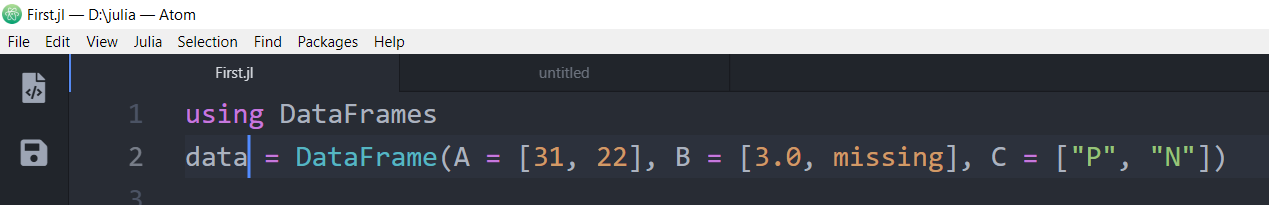Output: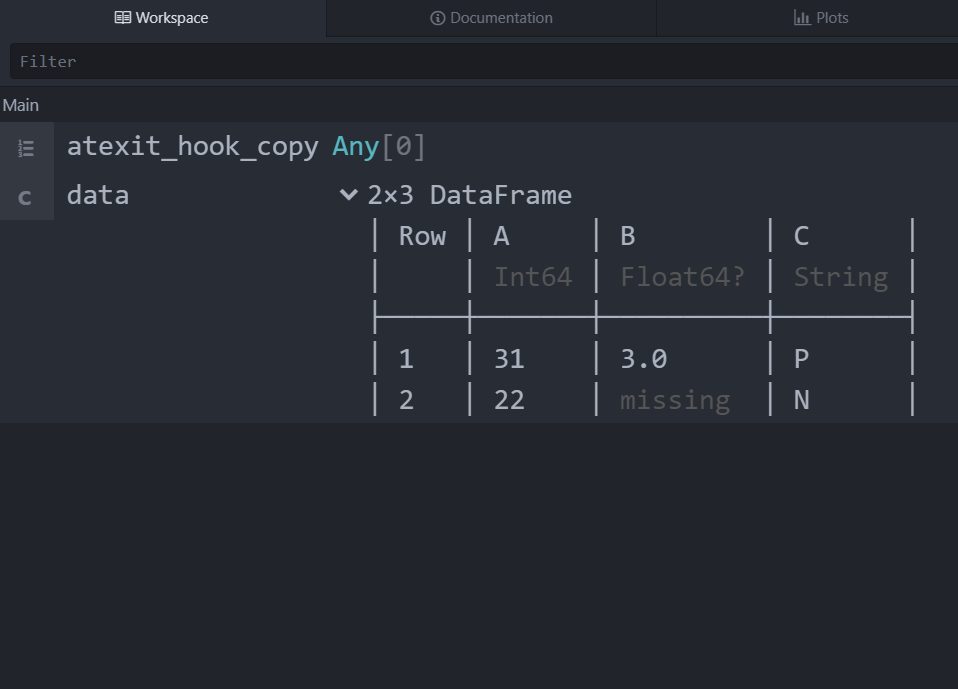Now that we have created a DataFrame of order 2×3 which is stored in a variable called “data” and we can also perceive that the data is stored in a tabular composition. Let us understand the structured data inside the DataFrame.

Here columns A, B, and C act as keywords.

• Column “A” comprises integer values.
• Column “B” includes Float and a missing value.
• Column “C” holds a String character.

#### Operations on DataFrames

Now let us comprehend some of the operations in Julia.

## Python3

 `# Julia program to create a Data Frame` `# Loading Library``using DataFrames` `# Creating a Data frame``data2 ``=` `DataFrame(A ``=` `1``:``8``, B ``=` `["M", "F", "F", "M", "F", "M", "M", "F"],``                           ``C ``=` `["T", "F", "T", "T", "F", "T", "F", "F"])`

Here, another Dataframe is created and stored inside a variable “data2”.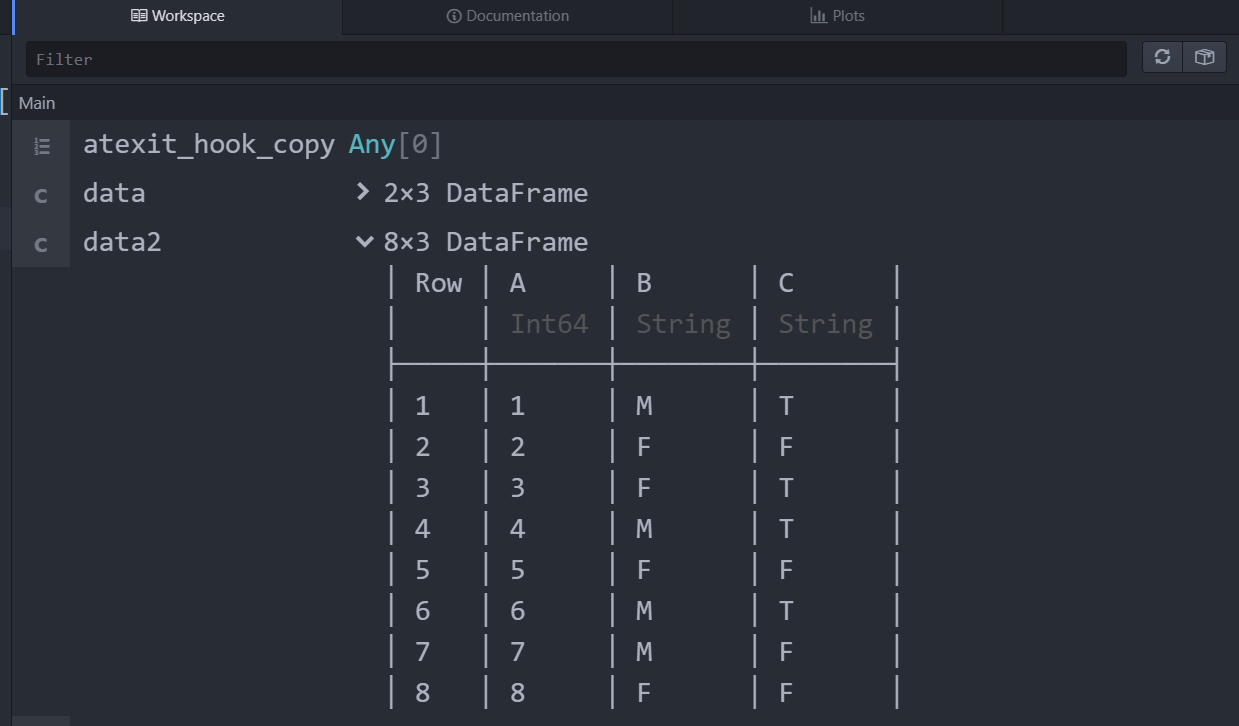This operation demonstrates the head part of the DataFrame

## Python3

 `# Demonstrating Head of Data Frame``x ``=` `head(data2)`

For the above DataFrame created, we have performed the head operation. It displays the topmost values in the dataframe. Output: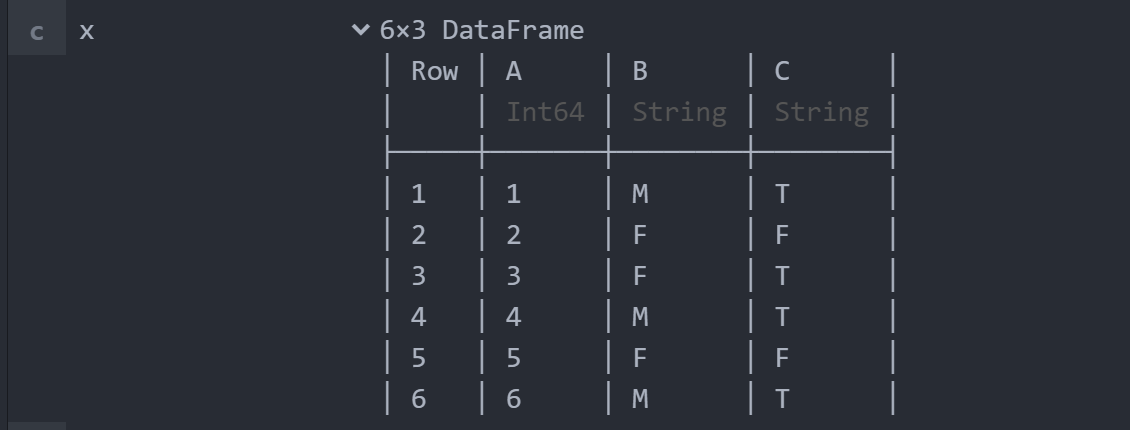Tail Function

This operation displays the tail part of the DataFrame.

## Python3

 `# Demonstrating Head of Data Frame``y ``=` `tail(data2)`

For the above DataFrame created we have performed the tail operation. It displays the bottom-most values in the data frame. Output: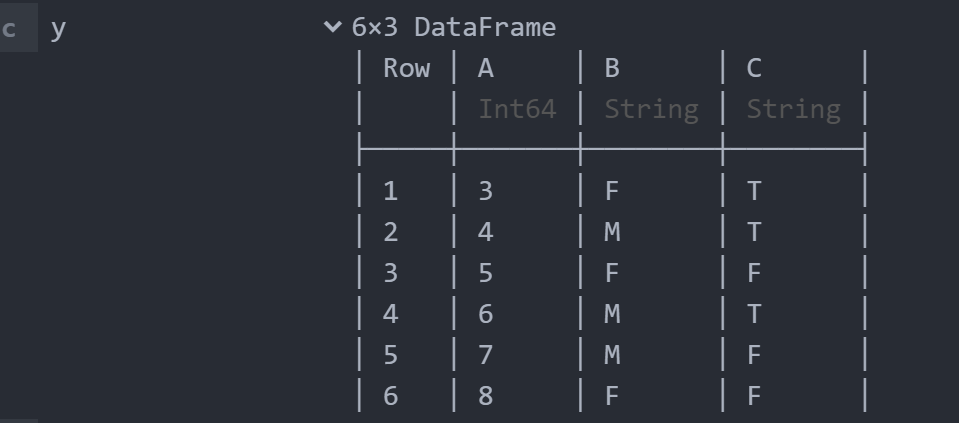Row and Column operations

## Python3

 `# Printing specific rows and columns` `# Using Row and column operation``z ``=` `data2[``1``:``4``, :]``s ``=` `data2[``1``, :]`

The above code represents the row and column operations.

• The number which is to the left of “, “(comma) represents the number of rows to be included.
• The number which is to the right of “, “(comma) represents the number of columns to be included.
• In the first variable(i.e, z), we are accessing the rows ranging from 1-4. The important part here is towards the right of the comma operator there’s a colon(” : “) which indicates that all columns to be included.
• In the second variable(i.e, s), we are locating only the first row with all columns included.

Output: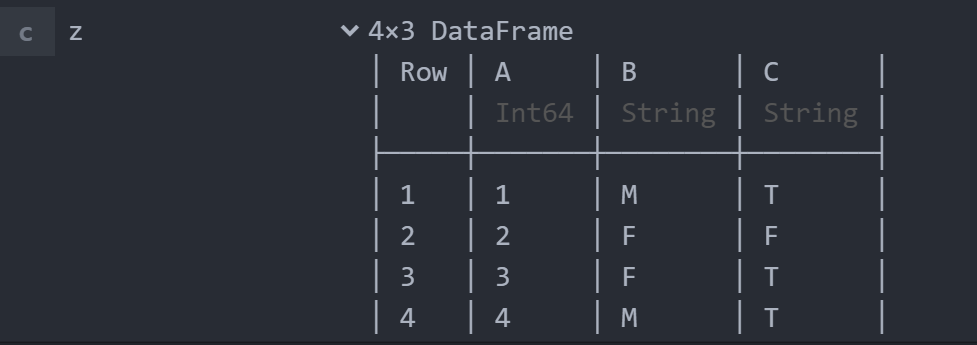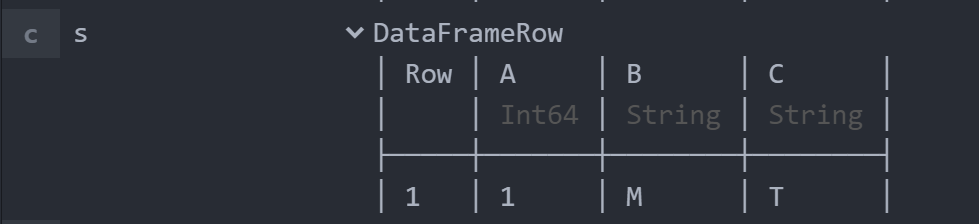#### Reshaping a DataFrame in Julia

Reshaping Dataframe includes the stack function. The data is manipulated and retrieved in a more precise form.

## Python3

 `using DataFrames``data3 ``=` `DataFrame(number ``=` `[``1``, ``2``, ``3``, ``4``, ``5``, ``6``, ``7``, ``8``],``                  ``id1 ``=` `[``1``, ``1``, ``2``, ``2``, ``2``, ``3``, ``3``, ``3``],``                  ``type` `=` `["dog", "dog", "cat", "cat",``                          ``"cat", "fish", "fish", "fish"])`

In the above code, we created a dataframe having 3 columns and 8 rows with numbering given in “number” column and “id” for each “type” given (i.e, id “dog” = 1, id “cat” = 2, id “fish” = 3) in the id1 and type column respectively. Output: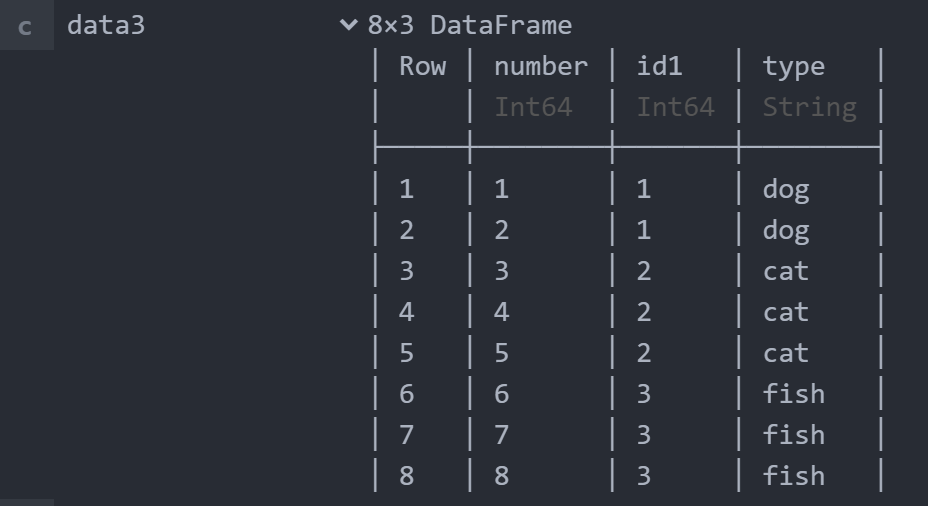Let us look at the reshaping property using the DataFrame declared above by using the “stack” function.

## Python3

 `# Reshaping the Data Frame``a ``=` `stack(data3, [:``type``, :id1], :number)`

Output: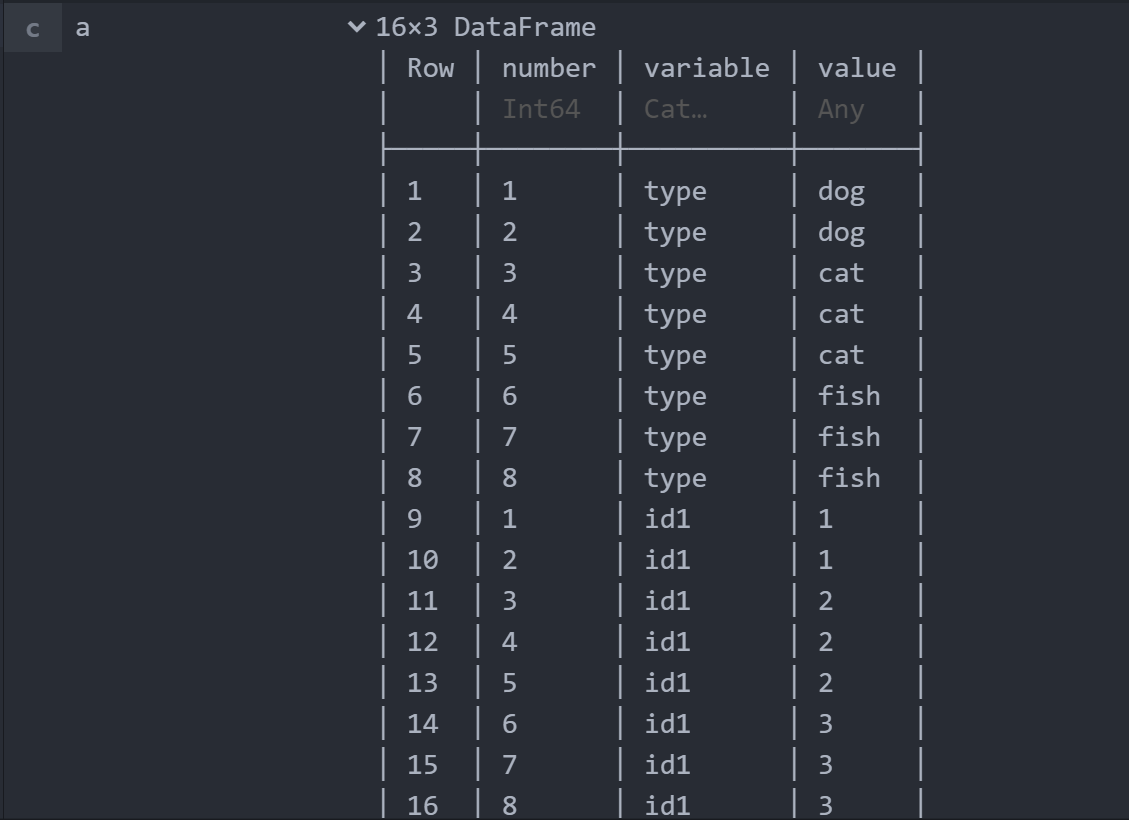By comparing two of the above pictures, we come to the conclusion:

• Inside the Stack function, we need to pass the variable in which our DataFrame is stored
• Hence, we are performing the reshape operation on our DataFrame created above and store in the variable “data3”
• Right after declaring the variable, leading towards the manipulating operation considering the “type” and the “id” columns displayed as strings in the row format.
• Hence repeating the number column values twice.
• We can now visualize the DataFrame is of order 16×3 after performing reshape operation using stack function.

#### Deleting Rows from a Data Frame

To delete rows in Julia we use a function named deleterows!(). It takes data frame name and row indices as arguments.

## Python3

 `# Deleting rows from a data frame` `# Calling deleterows!() Function``doc ``=` `deleterows!(data3, ``6``:``8``)`

Explanation:

• As we have created a DataFrame above and stored it in a variable “data3”
• Hence, we have performed the delete operation on that respective DataFrame.
• In the above code, we have deleted the rows 6 and 7 (n : (n-1)) by using the function deleterows! and stored the result in the “doc” variable.

Output: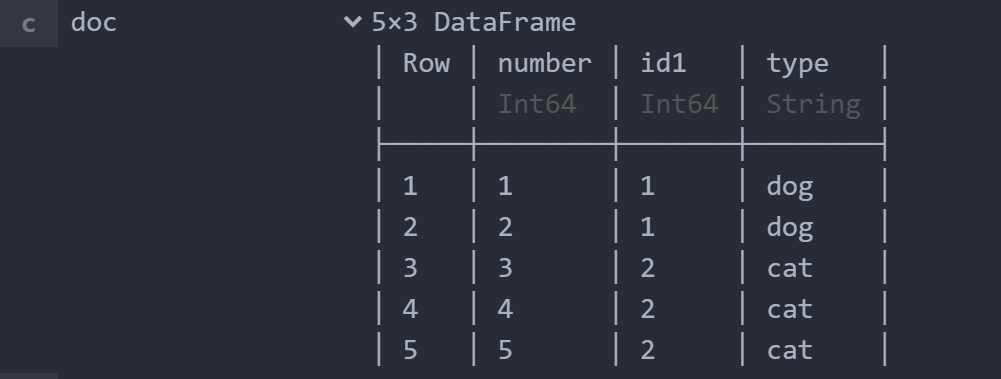Conclusion

Momentarily, we ultimately discovered what DataFrames really are in Julia and got to know all the procedures and done manipulating the data. In this article, we sophisticated mainly about reshaping the Data and the delete operation.

My Personal Notes arrow_drop_up